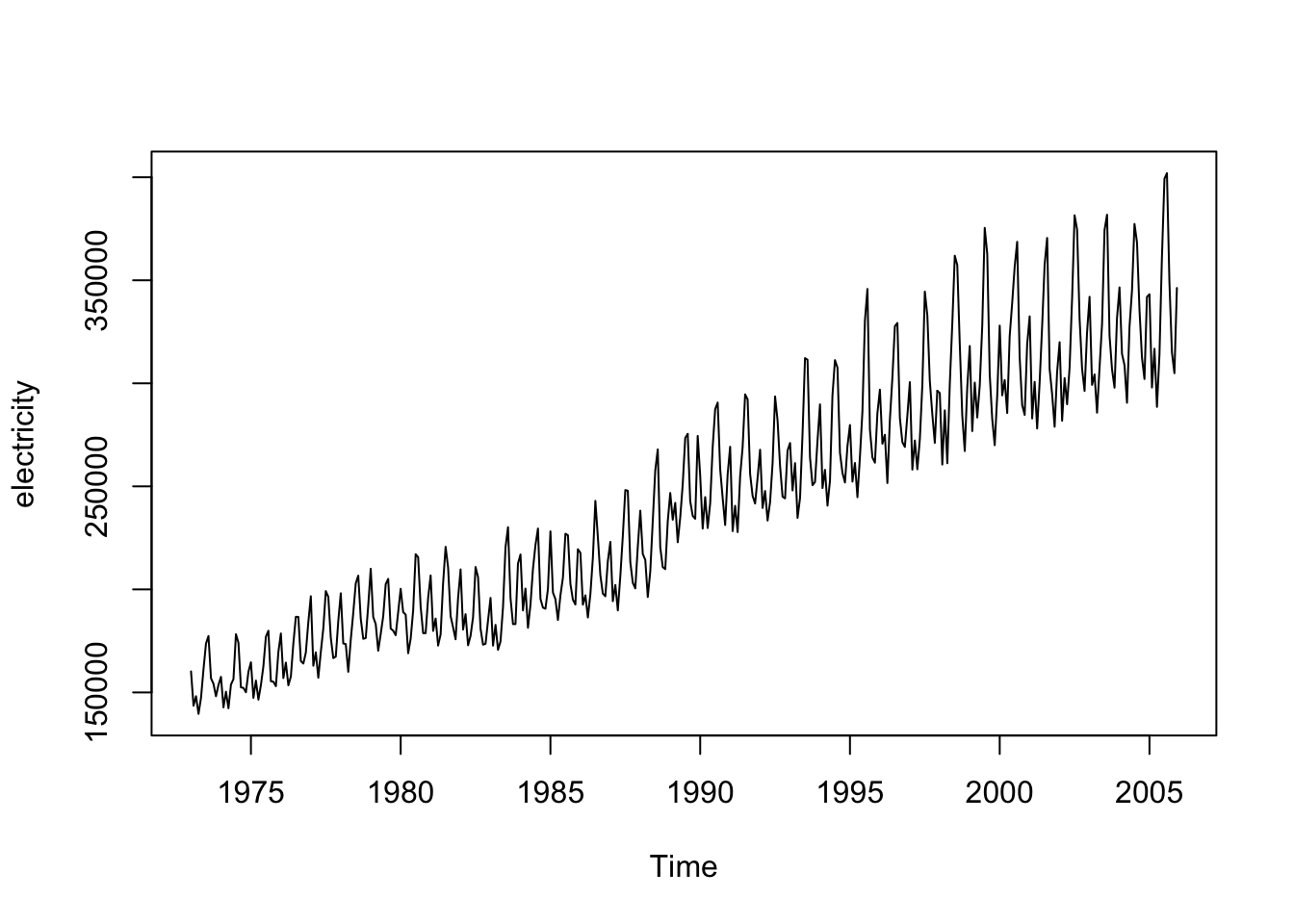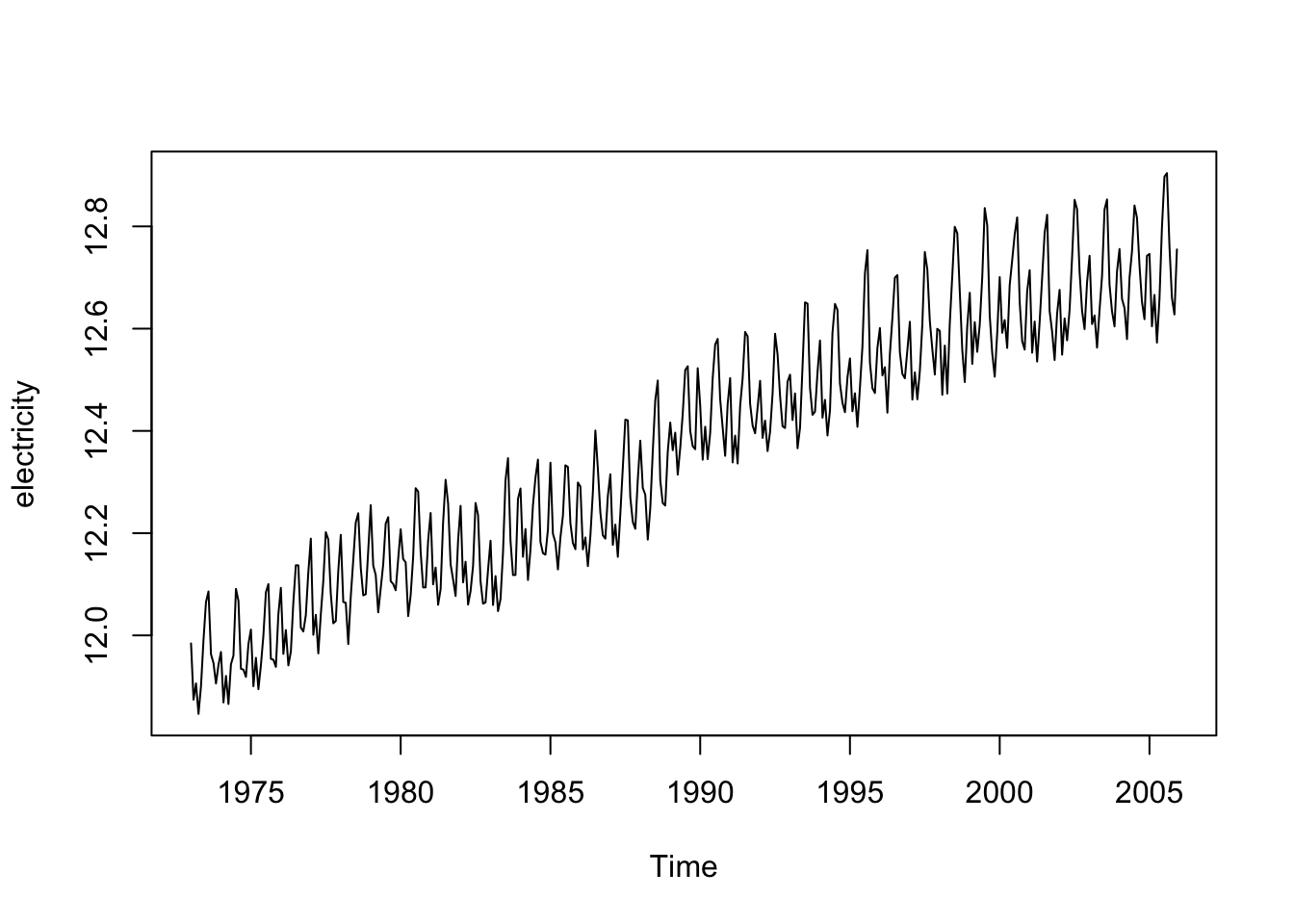### Load library “astsa” and set working directory

``````library(astsa)
library(fGarch)``````
``## Loading required package: timeDate``
``## Loading required package: timeSeries``
``## Loading required package: fBasics``
``````##
## Attaching package: 'fBasics'``````
``````## The following object is masked from 'package:astsa':
##
##     nyse``````
``# set your working directory``

### (a) By visually checking, decide what SARIMA models seem appropriate, i.e., specify p, d, q and P,D,Q in SARIMA model, ARIMA(p, d, q) ∗ ARIMA(P,D, Q)𝑠. Choose the most appropriate two models and explain your answer.

``````load("Electricity.RData")
plot.ts(electricity)````````````log.electricity = log(electricity)
plot.ts(log.electricity)````````````diff.log.electricity = diff(log.electricity, lag = 1)
plot.ts(diff.log.electricity)``````